## Biomedical Research

All submissions of the EM system will be redirected to Online Manuscript Submission System. Authors are requested to submit articles directly to Online Manuscript Submission System of respective journal.

Research Article - Biomedical Research (2017) Volume 28, Issue 5

## A novel approach for new-born authentication and verification using multibiometric features

Madhu M1, Moorthi M2*, Sathishkumar S3, Vimalagopalan TS4,5

1Rajiv Gandhi College of Engineering, Chennai, India

2Prathyusha Institute of Technology and Management, Chennai, India

3Department of Telecommunications, Asia Pacific University of Technology and Innovation (APU), Kuala Lumpur, Malaysia

4Formarly Deputy Director of Medical Service and Senior Civil Surgeon, Government of Tamilnadu, India

5Medical Officer, RYA Hospital, Iyyanavaram, Chennai, India

*Corresponding Author:
Moorthi M
Prathyusha Institute of Technology and Management
Chennai, India

Accepted date: September 23, 2016

Visit for more related articles at Biomedical Research

### Abstract

In this paper a novel approach for new born babies authentication and verification are done using multibiometric features, which are, mother’s fingerprint and baby’s foot print. The decision on recognition is done taken on fusion in which the feature vectors are extracted for query images independently, at matching score level, and are then compared to the database templates for each biometric trait. Based on the proximity of feature vector and template, each of the subsystem computes its own matching score. These individual scores are further combined together as a total score and are passed to the decision module. Thus, the multimodal system is proposed through finger and foot print fusion and recognition. The system proposed is tested using database images collected which comprise of different mother’s fingerprints and babies’ footprints. The overall system accuracy is found to be 96% with 0.001% and 1.23% false match rate and false non match rate respectively.

## Keywords

Biometric features, Fingerprint, Matching, Verification.

## Introduction

Biometrics is a wide area and highly researched topic to improve for identification and verification for security reasons. Biometric systems can be used as either identification or verification based on the application.

The usage of online footmarks over the other individualities similar to ear, face, fingerprint, and palm pattern for the babies is well conversed in . Biometric recognition of baby’s footmark feature which comprehends online image capture from two different kinds of background conditions and the shortcomings of offline method are also explained in . The knowledge gained from the unimodal system has been used to produce multimodal system which includes another biometric attribute. Hence, the identification of babies was carried out using serial mode of integration of the footprint of the baby and fingerprint of the mother . The retrieval and verification process was done to create the identity with N+1 computation.

Reliable verification schemes are required for various applications to verify the identity of an individual requesting the service. Examples of such applications include security for building access, computer systems, laptops, cellular phones and automatic teller machines. In the absence of vigorous verification schemes, these systems are susceptible to the tricks of a deceiver .

An overview of multimodal biometrics has been presented by Ross et al. and they also have suggested various levels of fusion, possible scenarios, and different modes of operation, integration strategies and design issues . Using the mixture of multiple classifiers significant amount of work has been carried out. For increasing the overall performance, most of such works emphasize on fusing ’weak’ classifiers . They have proposed a hybrid fingerprint matcher which fuses minutiae and reference point location. Ross et al. have proposed a methodology to tally level fusion . On face, fingerprint and hand geometry using product rule and coupla method Investigational results have been presented. From the investigation results it is found that both fusion rules show improved performance than individual recognizers. Guiyu has presented a novel fusion approach for individual identification using palm print and face biometrics . The work reflects the feature level fusion scheme. The tenacity of the suggested paper is to analyse whether the integration of face and palm print biometrics can attain greater performance which might not be possible by means of a single biometric indicator. In this feature vector fusion environment both Independent Component Analysis (ICA) and Principal Component Analysis (PCA) are considered. The performance has revealed that there is a significant improvement.

Currently the multi-biometric system is implemented in a more rigorous technique which involves the identification to be exploited fully to solidify a decision over the newborn identity claim.

## Proposed Method

The proposed system block diagram is as shown in Figure 1. The proposed system consists of three main stages, namely, sensor stage, image processing stage and transmission stage.

Sensor stage

Child footprint database: This database comprises of the foot legal permission from the Primary Health Center (PHC), which is one of the Indian government aided hospital. The data images are collected by wrapping a black cloth around the ankle within two consecutive days after the birth of the child. This is basically done, to provide high accuracy during the image segmentation process [9-14]. Figure 2 shows the sample newborn foot prints collected prints of the newborn new born child’s, captured with the help of a DSLR camera .

Mother fingerprint database: This database comprises of the newborn mother’s finger print, captured with the help of a USB finger print reader. Figure 3 shows the sample mothers finger prints collected.

Both the databases are collected from ten newborn-mothers consisting of eight footprints and eight fingerprints respectively, contributing to 80 biometric images, in total. Thus, 80 biometric images are stored with details such as mother’s name along with date and time of birth of the new born.

Image processing stage

This stage explains the development of the fingerprint and footprint algorithms using image processing as shown below.

Finger print processing: The finger print processing undergoes the following phases:-

Pre-processing: This is done in order to reduce noise and improve the overall visual appearance of the captured images. This further involves the following steps:

Finger print region masking: In this stage of pre-processing, unwanted or non-informative regions of the finger print are masked, as they do not contribute to the overall recognition.

Histogram equalization: This process is done in order to enhance the images obtained, so that the visual appearance in terms of contrast is better and can be used for any further operations.

Fast Fourier transform: This is taken in order to enhance the image further more. The histogram equalized image is divided into various small processing blocks. Each block is of 32 by 32 pixel matrix. Fourier transform of these blocks is taken as per the Equation 1,

Where, u=0, 1, 2, ..., 31 and v=0, 1 ..., 31 → (1)

The magnitude of the original block using FFT is given as,

FFT=abs (F (u, v))=|F (u, v)| → (2)

The enhanced block is given as per the Equation 3,where F-1(F(u, v)) is given by Equation 4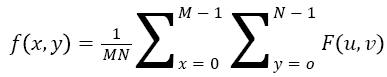Where x=0, 1, 2, ..., 31 and y=0, 1, ..., 31. Thus, the image looks more enhanced, after applying FFT in a better way, thereby removing any spurious noises.

Adaptive thresholding and image binarization: First thresholding is done to detect the edges and non-edges, by giving values of 0 and 1 respectively to gray images. Hence the gray images are converted into binary images. Thus, by this process, the edges of the face are detected and highlighted in black, while white is used to highlight the non-edges. This results in an improved contour of the finger print image.

Image segmentation: It is a process in which a given image is divided into several non-overlapping regions. It basically extracts the ROI (Region of Interest), from the given background image. Thus, it is proposed methodology; it is used to detect the edges from the given Finger print images, for further processing. Image segmentation in this proposed method is implemented with the following algorithm, which is explained further.

Least square approximation using block direction estimation algorithm: This algorithm is developed in order to detect the lines of edges more accurately and clearly.

Step 1: Determine the gradient values along both the axis (gx) and (gy). This is done for each pixel of the blocks segmented already in the previous step. The gradients determined are given using Equations 5 and 6 for the horizontal and vertical directions respectively. This is accomplished by employing two Sobel filters as shown in Figure 4. Thus, Sobel filters are used to detect the line edges.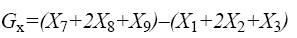→(5)→(6)

Step 2: The least square approximation for each pixel in each of the block is obtained using Equation 7.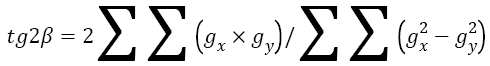→(7)

Step 3: After the direction of each block is estimated, those blocks without much significant information about the line edges and those which are not edges are discarded using Equation 8.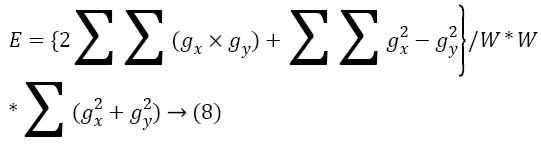→(8)

Where E is the certainty level and W is the estimation weight factor

Based on the certainty level E, decisions are taken. If E is below a certain threshold, then it is considered as the background block and hence discarded. Else it is considered as useful block. This is done for each block.

Morphological operations: This is done in order to eliminate the image areas that are not of effective and non-informative. Thus, only the remaining effective areas that are useful are sketched and bounded. Morphological operations such as OPEN and CLOSE are used. The OPEN operation is used to expand the segmented image and removes the peaks that have been introduced due to background noise, while the CLOSE operation is used to shrink the segmented images and eliminates any small cavities if so in the finger contour.

Feature extraction: This stage is used to extract main features from the segmented morphological finger print image. This involves edge thinning and feature marking.

Edge thinning: This process is done in order to eliminate the pixels of the line edges that are redundant in the finger print contour. The algorithm actually marks all the pixels that are redundant in each small window image of size 3 × 3, and finally, after several scans, all of those marked pixels are removed.

Feature marking: This is done to all the edge thinned images with an Id for each and is labelled using the morphological operation and is used to identify all the global points on the Finger print image.

For this an average inter edge width of D is estimated and this refers to the average distance between any two of the neighbouring line edges within the segmented morphological finger print contour. The procedure to calculate the width D is done by scanning a row of the edge thinned image and by summing up all the pixels values in that row results in a value equal to one. Then the row length is divided by the above summed value in order to determine the inter edge width. This is done for all other rows and columns and finally the average of all the inter edge width is determined to get the width D, which is more accurate.

Post processing: Post processing is done in order to remove false features if any, which occur due to insufficient illumination and this, is required as the pre-processing does not completely remove the noise of the finger print image. Also, the stages before introduce artifacts which further lead to false features, and if these false features are considered as genuine features, which will affect the accuracy of the final matching process of the finger print images. Hence, these false features must be removed in order to maintain effective finger print recognition. The following steps illustrated how these false features are removed.

Step 1: Let D be the average inter edge width. Now, when the distance between one edge branch and end point of the same edge is lesser than the width D and if the two features are in the same edges, then remove both of the edge points.

Step 2: When the distance between the two edge branches is lesser than the width D and if the two features are in the same edges, then remove both of the edge points.

Step 3: When the distance between the two edge branches is within the width D and if their respective directions coincide with a smaller angle variation comparatively, then this implies that the two edge end points are derived from the broken edge and considered as false feature, which are removed finally, as they are not of significant interest.

Step 4: Also, when the two edge end points are highly located within a short edge, whose lengths are lesser than the width D, then remove both of the edge points.

Thus, this overall process reduces the computational complexity involved, by removing all false features, which is an added advantage in the proposed method.

Feature matching: This is the final stage and is done by developing an alignment based matching algorithm which is explained as below:

Alignment algorithm

Step 1: First any one of the features of the image is selected and a distance measure of similarity is calculated between the two edges that are in association with the two feature points that are referenced.

Step 2: When the similarity measured is greater than the set threshold value, then transform each set of existing feature into a new co-ordination system, with the origin as the referenced point and x axis coinciding with the referenced point direction.

Thus, the matching is done between the query and the template images.

Relax matching algorithm: This algorithm is used to count the number of matched feature points, by comparing two feature points that are having almost the same direction and position. This is done to the transformed feature points obtained in the alignment algorithm. This is done by calculating the matching score, which is defined as given by Equation 9.

Matching score=No.of matched feature points/Max (No.of feature points (Image 1, Image 2) → (9)

Thus, the finger print algorithm is summarized in few steps as follows:

1. Convert the given RGB finger print image into gray.

2. Enhance it and perform FFT.

3. After FFT, perform image segmentation.

4. Determine the image gradients Gx, Gy.

5. Estimate the orientation of the local ridge, at each and every point of the image.

Foot print processing: The foot print processing undergoes the following steps:

Pre-processing: This is done in order to reduce unwanted background noise and improve the overall visual appearance of the captured images as before. Hence, first the RGB image is converted into a gray scale image. This is followed by image segmentation, morphology and ROI extraction as explained in the finger print image processing.

Feature extraction: This is done by employing a Gaussian filter to extract the discriminating features, based on the texture information of the given foot print images. The extracted information is further saved as different patterns in the database, respectively. Finally a similarity measure is performed to find the best match between the query and the trained pattern. A high similarity score reveals that the foot print belongs to the correct same infant.

Thus, the foot print algorithm is summarized in few steps as follows:

1. Convert the given RGB finger print image into gray.

2. Determine hundred strongest feature points.

3. Determine the putative matched point and display them.

4. Locate the object and apply transforms to eliminate the outliers.

5. The estimated transform to be applied is given using Equation 10. This helps to localize the required objects in the scene.

Transform matrix, T=R × B → (10)

Where,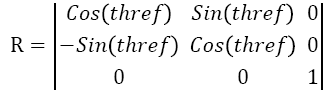, andwhere, I=index of reference points; M=Extracted features.

6. Finally display the matching points and send SMS, if M1>1000 and M2>1000

Score level fusion stage: In the proposed method, fusion takes place at the match score level, as it is easy to fuse and due to the fact that all the commercial devices available do provide access to scores [16-18]. The finger print score of the mother obtained in the previous stage ranges in the interval between 0 and 1000, while the foot print score ranges between 0 and 1. Since both the scores are of different ranges, the finger print score is normalized using the min-max normalization, which is then transformed to a common interval between 0 and 1. When the matching scores {Sk}, k=1, 2,.., n , then the normalized scores are given by Equation 11,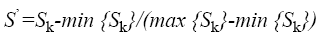→(11)

where S’ is the normalized score

After normalizing the finger print score, both the scores are now fused based on the sum rule fusion given using Equation 12.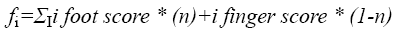→(12)

where n is the matcher weight, which is multiplied to each of the matcher, such that the sum is equal to unity, and i = 1,2,3,…, I is the number of users.

Based on the preliminary results obtained, equal weights are considered to be used. Thus, the weighted matching fusion rules are used . Finally, the fused score fi is compared to a pre-determined threshold (t). If fi ≥ t, then the new born baby is declared to be the kid of the corresponding mother, else declared to be an imposter.

Transmission stage

This section explains the communication module involved in the transmission of the data, Global System for Mobile communication-GSM. The outcome of the fused system is indicated by an authorized person to the hospital child care in charge via mobile application.

## Experimental Results

The proposed method is tested with the database images collected. The database is collected from the Primary Health Center, Medavakkam, Chennai, India. A total of 240 images have been collected, from 40 new-borns. Out of which six images are from the right foot of each of the new born babies and corresponding finger prints from the respective mothers is also collected. Thus 240 foot prints and 240 finger prints have been collected resulting in a total of 480 images as database images. One image is used to test the system performance, while 40 images have been used for training.

Figure 4 shows the Foot print images undergoing various stages of the feature extraction process. Figure 5 shows the Finger print images undergoing the edge detection, feature extraction, putatively matching pints followed by matched inlier points.

Figure 6 shows the finger print matching process with the various stages undergone with the GUI (Graphical User interface) developed. Figure 7 shows the system interfaced with the mobile application showing the identification of the mother with the respective new born.

ROC analysis

The proposed system’s performance on recognition of the finger print and foot print, when fusion is performed is determined in terms of the ROC (Receiver Operating Characteristics) analysis. Figure 8 shows the ROC curve so obtained. Here, the curve is obtained by taking the False Match Rate (FMR)) along the x-axis and False Non Match Rate (FNMR) along the y-axis. FMR is basically equivalent to the False Acceptance Rate (FAR) and FNMR is the False Rejection Rate (FRR).

The false acceptance is the one when the imposter score exceeds the threshold and false rejection is the one when the genuine score falls below the threshold. EER (Equal Error Rate) refers to the point in the ROC curve, where the FMR equals the FNMR. It could be observed that the EER is less than 0.1, indicating a better performance of the proposed system. But the Decision Threshold (DT) is fixed to be at 0.47 of the similarity score, for high security application. At this value of DT, the FMR is 0.01% and the FNMR is 12.3%. The inference is that FMR 0.01% guarantees that for 10,000 attempts to access by imposters, only one of them could be successful, which is highly acceptable rate. However, with 12.3% FNMR, twelve percent of the genuine attempts of matching may go wrong, but can be repeated.

## Conclusion

In this paper, the novel approach using multi modal biometric system is proposed by integrating the new born foot print and respective mother’s finger print for identification and verification, using the fusion technique. A matched score level for fusion is developed, in which sum rule based fusion is chosen and tested with the 480 database images. Based on the results obtained an ROC curve analysis is evaluated and inferred that the FMR achieved is 0.01% with 12.3% FNMR, leading to a 96% accuracy. The outcome of the fused system is indicated by an authorized person to the hospital child care in charge via mobile application. Thus, the proposed system is low in cost compared to other existing systems.